# Spiral

### From DT Online

##### Description

An Spiral is a line traced by a point which starts at a centre then gets progressively further away as it travels around it. There are many different ways of drawing spirals each with different mathematical properties.

A vynil record groove is a good example of a spiral (but the early, antique phonograph cylinders follow a helix. Spiral staircases are also in fact helixes and not really spirals at all (!) but 3D spirals do exist, examples of which include the springs commonly used as a battery contact in torches and some snail shells).

##### Constructions
 Drawing a Common Spiral It is possible to draw a spiral-like curve using only semi-circles. This is not a true spiral but is often sufficient for illustration purposes. Start by drawing a line of any length then describe a small semi-circle somewhere near the centre. Use the diameter of the first semi-circle as the radius for a second sem-circle described from one end of the first one. Draw a third semi-circle from one end of the second with its radius set to the diamater of the second semi-circle. Repeat this procedure until you have enough turns or a large enough spiral for your purposes.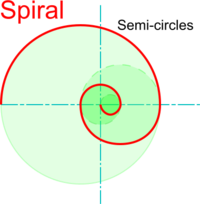Constructing an Archimedean Spiral Named after the famous ancient Greek mathematician, Archimedes, this spiral is: the path traced by a point as it moves away with constant linear velocity, from the centre of a circle which is rotating with constant angular velocity. A line produced by a point following a set of rules in this way is known as the locus of a point (plural is loci). Start by drawing a number of equi-spaced concentric circles (say, 12) and divide one complete revolution into the same number of equal parts. The intersection between the circle from point 1 on the line and radial line 1 provide point 1 on the spiral. Plot all other points in this way and draw a smooth curve through them (or use French Curves or a flexible strip for example)Constructing Spiral using Golden Rectangles A Golden Rectangle is one in which the sides are in a particular proportion known as the Golden Ratio or Golden Section. A good way of approximating this ratio is to use the Fibonacci series which is created by making each number the sum of the two before it - i.e. 1,1,2,3,5,8,13,21, etc. The further along the sequence you go: the nearer you get to the Golden Ratio. It is a property of a Golden Rectangle that when a square is added to its longest side, another, larger, Golden Rectangle is created. Start by drawing a square of any size (but for ease, make its length of side divisable by a number on the Fibonacci series - say,5) Draw a rectangle where one side is the side of the square and the shorter side has a length proportional to the number below it on the Fibonacci series (say, 3). Draw a large square from the side of the first square plus the shorter side of the rectangle (length now, say, 8) and repeat the process. An approximate spiral can be created by drawing quarter circles, or quadrants, in each of the squares as shown.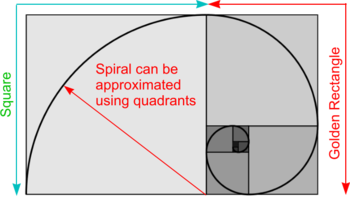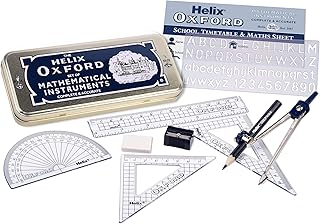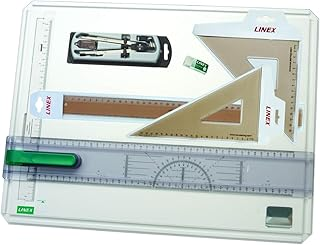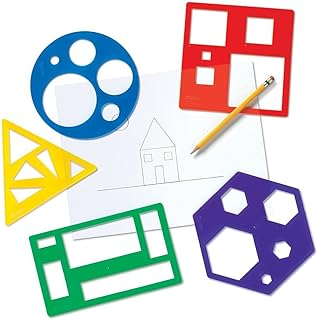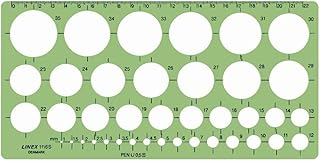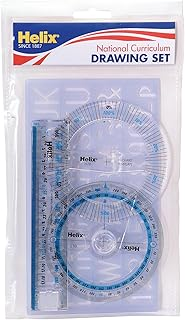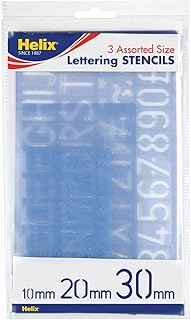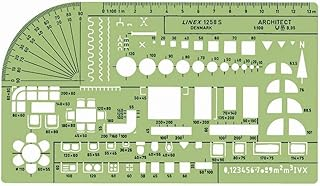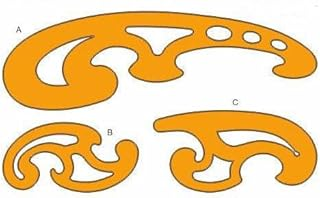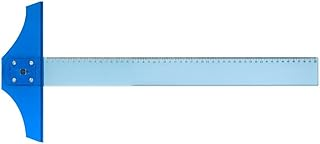Helix Oxford Maths SetLinex Student Saver BundleHelix Precision Plus Drawing SetPrimary Shape Template SetLinex Circle TemplateHelix National Curriculum Drawing SetHelix 3 Piece Stencil SetLinex Universal Architects TemplateFrench Curve Transparent TemplateT-Square